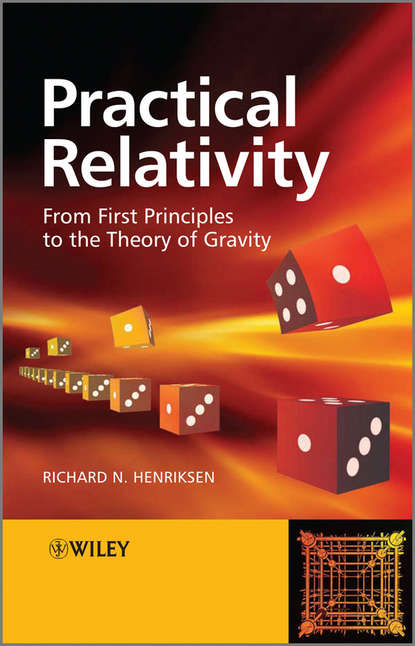﻿ Practical Relativity. From First Principles to the Theory of Gravity (Richard N. Henriksen) – скачать в fb2, epub, pdf на FenzinСкачать полностью

# Practical Relativity. From First Principles to the Theory of Gravity

Электронная книга
Добавлено 21.02.2020
Год издания: 2018
Аннотация

The book is intended to serve as lecture material for courses on relativity at undergraduate level. Although there has been much written on special relativity the present book will emphasize the real applications of relativity. In addition, it will be physically designed with the use of box summaries so as to allow easy access of practical results. The book will be composed of eight chapters. Chapter 1 will give an introduction to special relativity that is the world without gravity. Implications will be presented with emphasis on time dilation and the Doppler shift as practical considerations. In Chapter 2, the four-vector representation of events will be introduced. The bulk of this chapter will deal with flat space dynamics. This will require the generalization of Newton's first and second laws. Some important astronomical applications will be discussed in Chapter 3 and in Chapter 4 some engineering applications of special relativity such as atomic clocks will be presented. Chapter 5 will be dedicated to the thorny question of gravity. The physical motivation of the theory must be examined and the geometrical interpretation presented. Chapter 6 will present astronomical applications of relativistic gravity. These include the usual solar system tests; light bending, time delay, gravitational red-shift, precession of Keplerian orbits. Chapter 7 will be dedicated to relativistic cosmology. Many of the standard cosmological concepts will be introduced, being mathematically simple but conceptually subtle. The concluding chapter will be largely dedicated to the global positioning system as an engineering problem that requires both inertial and gravitational relativity. The large interferometers designed as gravitational wave telescopes will be discussed here.

Полная версия
﻿
Отзывы о книге Practical Relativity. From First Principles to the Theory of Gravity
Нет отзывов
Добавить отзыв

Чтобы добавить отзыв Вам нужно зарегистрироваться или авторизироваться